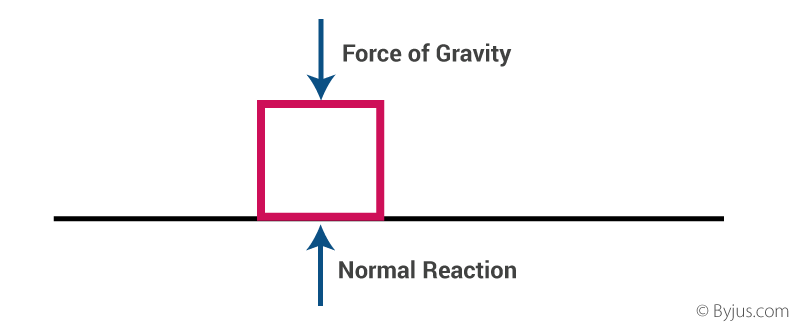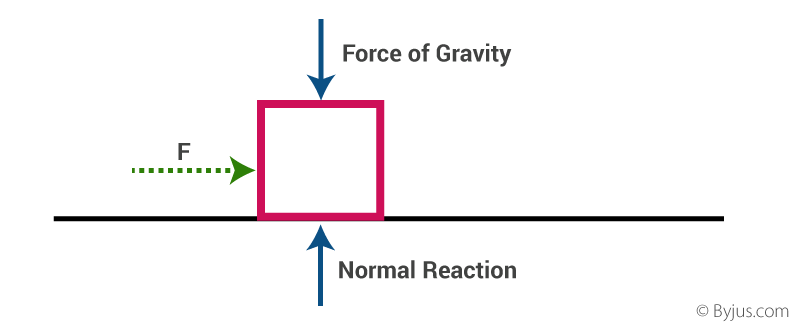top of page
Search
•Scholenberg

# NEWTON'S FIRST LAW IN DAILY LIFESIR ISSAC NEWTON, (born January 4,1643) in Woolsthorpe, England - (died March 31,1727) in London, England. He was an English Physicist and mathematician, and was a culminating figure in the Scientific revolution of the 17th Century. He made many great discoveries in the field of physics and mathematics, and is one of the most renowned scientist of all time. He made many discoveries in various fields some of them are as follows:

• The Laws of MOTION

• The Law of GRAVITATION

• Discoveries in Optics

• The Law of COOLING

• Discoveries in Mathematics

• Reflecting Telescope

• The Perfect Coin

NEWTON'S LAWS OF MOTION• Sir Issac Newton worked in many area of mathematics and physics. He developed the theories of gravitation in 1666 when he was only 23 years old and then later in the year 1686, he presented these laws of motion in “Principia Mathematica Philosophiae Naturalis.”• These laws of motion explain the relationship between a physical object and the forces acting upon it.

• Understanding these laws provide us with the basic information about modern physics.

• By developing these laws Sir Issac Newton revolutionized Science. It was after this that Newton's Laws along with Kepler's Laws explained why planets move in elliptical orbits rather than in circles.

NEWTON'S FIRST LAW OF MOTION• It is also referred to as "LAW OF INERTIA".

• Newton's First Law of Motion states that "An object at rest remains at rest and an object in motion remains in motion at constant speed and in a straight line unless acted upon by an unbalanced force."

• So it basically explains that any object at rest or in uniform motion in a straight line, will tend to stay in the same state, as long as no external force acts upon it.

• These tendency of any object to resist changes in a state of Motion is called Inertia.

• If there is no net force acting on the object i.e. if all the external forces cancel out each other , the object will maintain the same velocity, and if the velocity is zero, the object remains at rest.MATHAMETICAL EXPRESSION FOR NEWTON'S FIRST LAW OF MOTION:here V is the velocity of the object

t is the time

F is the force

This can also be easily understood by a real life example as follows:

1. Marble Balls On A Ramp• Imagine you have three ramps that are infinitely long and infinitely smooth.

• You let a marble roll down the first ramp, which is set at a slight incline. The marble speeds up on it's way down the ramp.

• Now you give a gentle push to the marble going uphill on the second ramp. It slows down as it goes up.

• Now consider a third ramp which is a middle state between the first two conditions, so it is a ramp which is perfectly horizontal. It will neither speed up nor slow down, in fact it should keep rolling forever.

2. Forces Acting On A Block

• Let us examine the forces acting on a block.• The forces acting on a block are the forces of gravity and the normal reaction by the surface.

• There is no force acting on it in the horizontal direction. Since the forces in the vertical direction are equal to each other in magnitude, they cancel each other out, and hence there is no external force on the block.

• Since this block is at rest, we can say that it confirms Newton’s first law of Motion.• Now, if we apply a constant force F on the block in the horizontal direction , it will start moving with the same constant acceleration, in the direction of the applied force.

• Thus, the first law of motion is confirmed again.

3. SUDDEN CHANGE IN MOTION OF BUS• When a bus starts moving all of a sudden, the head of the passenger sitting in the bus strikes the back of his seat.

• This can be explained on the basis of the first law of motion.

• The lower part of the body of the passenger remains in contact with the body of the bus, but the upper part is free. When the bus takes a sudden start, the bus comes in motion and so the lower part of the body, but the head and upper part of the passenger’s body not in contact with the bus, tend to remain at rest and thus, jerked backwards.

• Similarly, we can explain the jerking of passengers in the forward direction of a bus, when the moving bus stops all of a sudden.

So, we can thereby conclude that Newton's First Law Of Motion has great significance in our day-to-day life as we knowingly or unknowingly apply it in various situations of life and predict further motions related to ourselves or other prospects, resulting in better life quality.